Physics Tutor Series: Learning By Example

2005

This is a 24 video collection

In This SeriesVelocity and Acceleration In One Dimension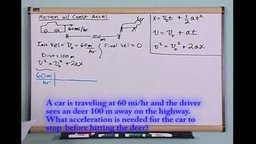Equations Of Motion In One Dimension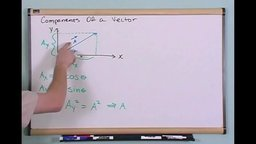Scalars and Vectors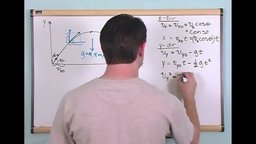Projectile Motion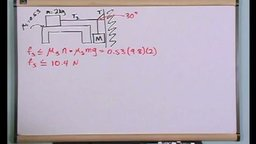Newton’s Laws Of MotionNewton’s Laws Of Motion With Friction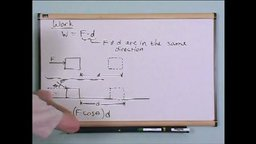Work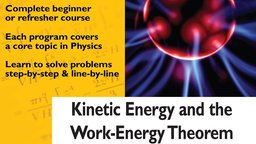Kinetic Energy and Work-Energy TheoremPotential Energy and Energy Conservation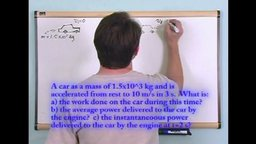Power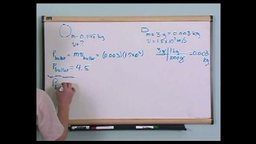Momentum and Impulse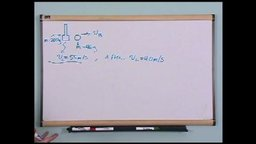Conservation Of Momentum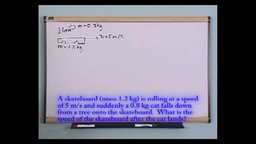Inelastic and Elastic Collisions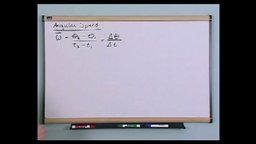Angular Speed and Angular AccelerationRotational Equations Of MotionTangental Speed and Centripetal Force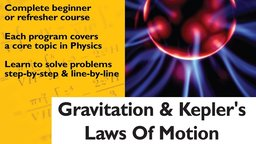Gravitation and Kepler’s Laws Of Motion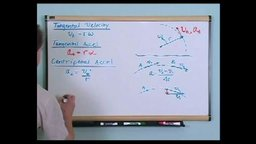TorqueRotational Equilibrium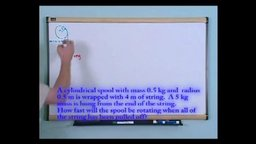Angular Acceleration & Moment Of Inertia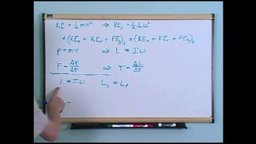Angular Momentum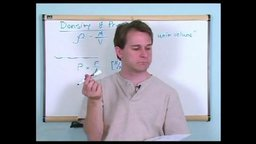Density and PressureBernoulli Equation